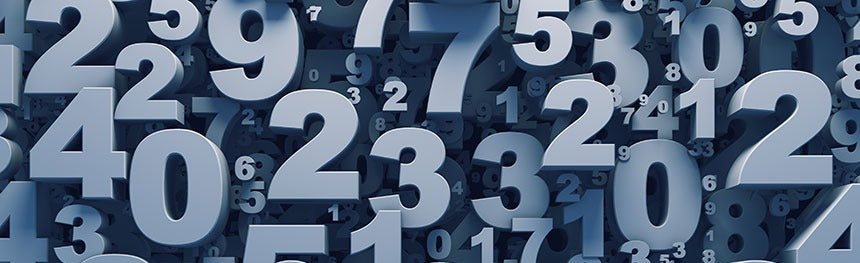# Warrior Wednesday 05.19.21 - Macro Math

The term 'macro' is short for macronutrients. These are the big nutritional building blocks that humans need to function and thrive: protein, carbs, and fats. Some people include fiber, but that typically is considered a sub-category of carbohydrates. You may hear of people "counting macros" or "counting calories." They are tracking their ingestion of these macronutrients throughout their day.It involves some math. Simple math, but with a few steps so sometimes it can get confusing.

First, lock this down in your memory:

1g of protein = 4 calories

1g of carbs = 4 calories

1g of alcohol = 7 calories (**Not technically a macronutrient but does hold caloric value**)

1g of fats = 9 calories

Second, we need to figure out how many calories we need to ingest on a daily basis. How you determine your total number of calories is honestly a lot of trial and error. Use a trusted macro calculator and it will give you a rough idea (Google 'Macro Calculator'), then you need to track your weight to see if you are gaining/losing/maintaining your weight week over week at those specific calories. Tracking weight ONCE PER WEEK is very important in determining how your caloric intake is working for you. If you are gaining weight, you are eating too much OR your activity levels are too low. If you are losing weight, you're not eating enough. If your weight stays flat, you are eating just enough calories to maintain your current situation.

For this example, let's use a round number of 2000 calories.

Let's hypothetically say your coach gives you a macro breakdown of 30% protein, 35% carbs, and 35% fats. Our math would look like this:

Protein - 30% = 600cal (2000/30=600) = 150g (600/4=150) Carbs - 35% = 700cal (2000/35=700) = 175g (700/4 = 175) Fats - 35% = 700cal (2000/35=700) = 78g (700/9 = 77.7778)

You can manipulate that as well. If it is given to you in grams then it would look like this:

Protein - 150g = 600cal (150*4=600) = 30% ((150*4)/2000=0.3) Carbs - 175g = 700cal (175*4=700) = 35% ((175*4)/2000=0.35) Fats - 78g = 700cal (78*9=700) = 35% ((78*9)/2000=0.35)

And in calories:

Protein - 600cal = 150g (600/4=150) = 30% (600/2000=0.3) Carbs - 700cal = 175g (700/4=175) = 35% (700/2000=0.35) Fats - 700cal = 78g (700/9=77.7778) = 35% (700/2000=0.35)

There is no perfect ratio of macronutrients for the masses. Everyone is different and everyone handles food differently. Your best course of action is to track your food intake. Talk to your coach, or use a macro calculator to estimate your macro ratio, track for 2 weeks, and see what happens. You can make adjustments from there.

Macro math.